Economics Tutors | Micro, Macro & Econometrics Tuitions | EconTutors

# Econometrics Tutor in London and online

## OLS estimator by our econometrics tutor in London and online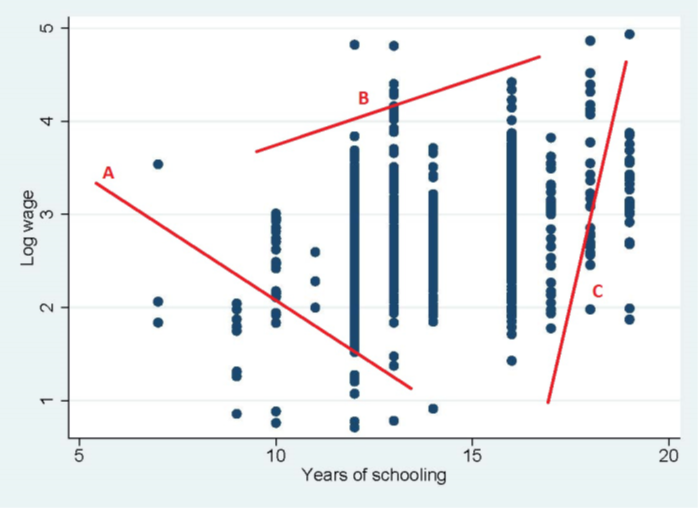Scatter plot for finding the best fit line.

#### OLS best fit line

The objective is to find the best fit line that goes through the scatter diagram. In this online tutorial, our econometrics tutor online in London works through OLS derivation.

1. The relation between log wage and eduation does not look like the regression line one would imagine.
2. The points are not randomly scattered, but seem to have a slight upward drift.
3. This relationship is not as exact as one would hypothesize. Regardless, we need to measure this relationship based on actual data.
4. We also need to be cognizant that education is not the only factor that determines an individual’s wage – there is obviously several other factors like experience, personality, soft-skills, industry type, job riskiness, etc.
5. To reflect these other factors, we add a disturbance term in our model.
6.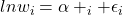With the disturbance term, the relationship between wages and education is no longer deterministic. It is stochastic. “Stochastic” comes from the Greek “stokhos”, meaning a target or bull’s eye. A stochastic relationship is not always “right on target” in the sense that it predicts the precise value of the variable being explained. In practice, however, economic relationships are rarely exact. If we could collect data on income and consumption for many different households, it is unlikely that all households would lie exactly along the line drawn above.
Firstly, income is not the only determinant of consumption (the model is a simplification of true economic behaviour). And secondly, there is randomness in human behaviour such that two people on the same income do not necessarily consume the same amount.
To reflect that the economic relationship is not exact, we transform our deterministic model into an econometric model by adding a disturbance or error termso that we get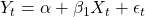Typically, we add the error term for three main reasons.

1. Omitted factors influencing the dependent variable
2. Measurement error in the dependent variable
3. Inherent randomness

Suppose that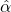and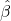are the estimators for the unknown population parameters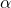and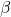.
The termis a random variable, also called a stochastic variable. It is used to represent all of the factors that affect consumption that we have not included explicitly in the model (maybe because they are variables that cannot be measured or observed) as well as the fact that there is inherent randomness in people’s behaviour.

#### Deriving estimators for OLSandA “good” estimator should be one that generates a set of estimates of the parameters that makes these residuals “small”. Most introductory econometrics classes start with the idea explained above, and proceed to deriving an estimator forand, typically deploying the ordinary least squares technique (OLS).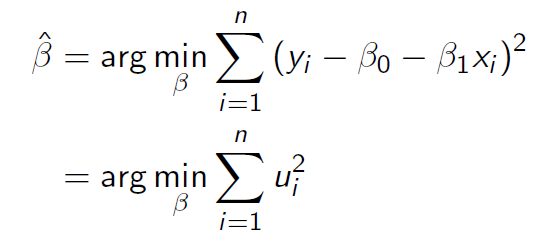OLS uses the technique of minimizing the sum of the squared residuals. The idea is that the best estimator is the one which fits a line that has the smallest possible residuals around this best fine line. Therefore, we write out the residual, which is simply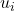or some books use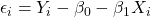. We then sum up all the residuals after squaring them. Then we minimize this summation. These first order conditions that are used to derive OLS estimators are show below.

#### OLS first order conditions, also called the normal equations.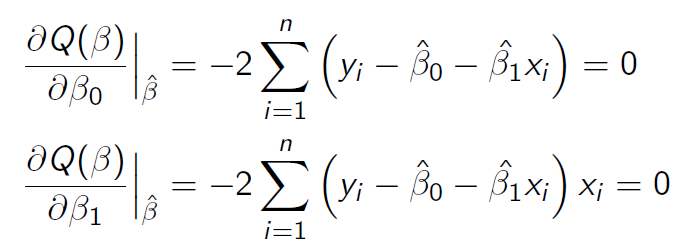Work online with our expert econometrics tutor online or in London to go over these concepts, derivations, and proofs to give you a solid understanding of econometrics theory and applications. Students at top universities are expected to derive these estimators on econometrics exams, and problem sets. Students should develop a comfort level in taking partial derivatives, and minimizing SSR to derivate an estimator.

#### OLS estimators

We obtain the following estimators by solving the above normal equations. These estimators ensure that the line (which is made up of an intercept and slope) is the best fit line. Again, what does it mean to have the best fit? A line that has the smallest possible residuals for all data points is considered the best fit line. Book a lesson with our econometrics tutor online London to learn about properties of these estimators.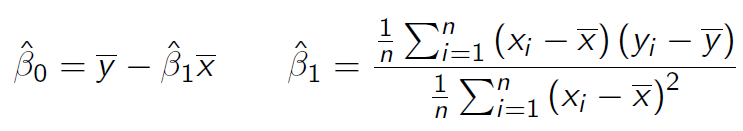Other popular courses:

Get Started

## See the #1 economics mentoring platform in action

Request a demo